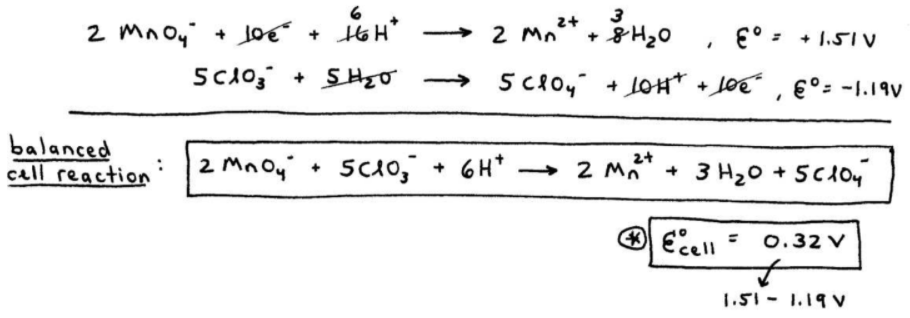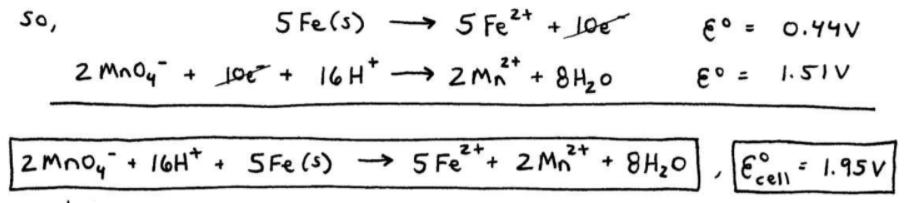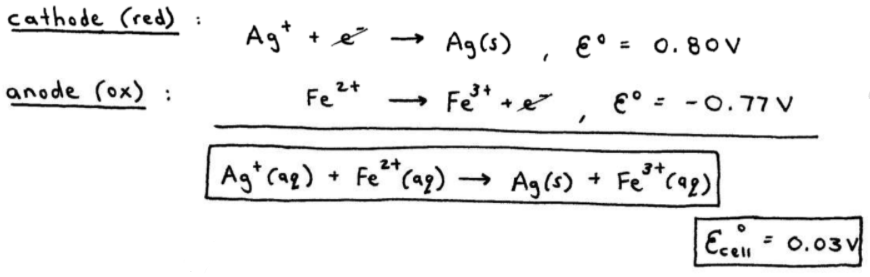# S17E3 - Galvanic Cell Examples and Balancing Half-Reactions

Today we're going to discuss three galvanic cell problems.

FYI:  galvanic cell  =  electrochemical cell  =  voltaic cell

----------

## Galvanic Cell Example #1

ex:  A galvanic cell is based on the following unbalanced equation:

MnO4-(aq)  +  8H+  +  ClO3-(aq)  →  ClO4-(aq)  +  Mn2+(aq)  +  H2O(l)

Give the balanced half-reactions, the balanced cell reaction, calculate E°cell, and give the cell's line notation.
_________

➞  half-reactions - use the Table of Standard Reduction Potentials from my previous video post to get:

(anode) oxidation,
ClO3-  +  H2  ClO4-  +  2H+  +  2e-  , E°ox = -1.19 V

(cathode) reduction,
MnO4-  +  5e-  +  8H+   Mn2+  +  4H2O   ,  E°red = +1.51 V

----------
➞  Then multiply each reaction through to get the number of electrons (10e-) to cancel:

2MnO4-  +  10e-  +  16H+   2Mn2+  +  8H2O   , E°red = +1.51 V
5ClO3-  +  5H2O    5ClO4-  +  10H+  +  10e-   ,  E°ox = -1.19 V

----------
➞  Eliminate and reduce to get the balanced cell reaction:

2MnO4-  +  5ClO3-  +  6H+ →  2Mn2+  +  3H2O  +  5ClO4-
cell  =  +0.32 V

See here...----------
➞  Finally, we can write the line notation:

Pt(s) | ClO3-(aq), ClO4-(aq), H+(aq) || H+(aq), MnO4-(aq), Mn2+(aq) | Pt(s)

or:What's "Pt" ? -- Platinum (Pt) is an inert metal that must be used as the conducting electrode when there's no metal(s) involved in the anode or cathode.

==========

## Galvanic Cell Example #2

ex:  "Completely describe" the following galvanic cell, given just its half-reactions:

Fe2+  +  2e-  Fe(s)   ,  E°red  =  -0.44 V
MnO4-  +  5e-  +  8H+    Mn2+  +  4H2O  , E°red  =  +1.51 V
_________

When "completely describing" a galvanic cell, we need to know how to:

➞ 1. write the overall cell reaction (E°cell > 0),

➞ 2. calculate E°cell,

➞ 3. designote anode and cathode,

➞ 4. show direction of electron flow and report ions in each compartment (line notation).

----------

Ok, looking at the two reduction half-reactions, the E°red  =  +1.51 V is probably written in the correct direction because we want E°cell to be overall positive (+ volts).

So we'll reverse the Fe / Fe2+ half-reaction...

(anode) oxidation,
5 x  [ Fe(s)    Fe2+  +  2e]   ,  E°ox  =  +0.44 V

(cathode) reduction,
2 x  [ MnO4-  + 5e-  + 8H+   Mn2+  + 4H2O ]  , E°red  =  +1.51 V

--------------------
➞  Now we can write the line notation:

Fe(s) | Fe2+(aq) || H+(aq), MnO4-(aq), Mn2+(aq) | Pt(s)

----------
➞  Finally, we can sketch the galvanic cell, designate the anode / cathode, and determine the direction of electron flow.

In a galvanic cell, electrons flow from anode to cathode!!==========

## Galvanic Cell Example #3

ex:  Given the following half-reactions, describe completely the galvanic cell:

Ag+  +  e-    Ag(s)  , E° =  + 0.80 V

Fe3+  +  e-    Fe2+  , E° =  + 0.77 V
_________

We want cell > 0, so "flip" the 2nd half-reaction to get:

(cathode) reduction,
Ag+  +  e-    Ag(s)   , E° =  + 0.80 V

(anode) oxidation,
Fe2+    Fe3+  +  e-   , E° =  - 0.77 V

Adding these two half-reactions together gives the following:----------
➞  Line notation:

Pt(s) | Fe2+, Fe3+ || Ag+ | Ag(s)
anode                                                    cathode

----------
➞  Sketch of the galvanic cell:==========

Coming up in my next post on SECTION 17 - Electrochemistry,